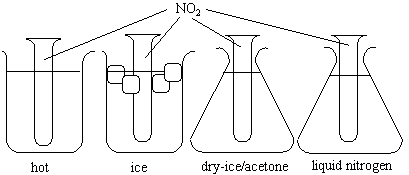## 21.1 The Effect of Temperature on the NO2/N2O4 Equilibrium

Chemical Concept Demonstrated: Temperature's effect on equilibrium

Demonstration:

 Four glass tubes are sealed with NO2 gas. The first tube is placed in a hot water bath. The second is placed in ice-water. The third is placed in a flask filled with dry-ice/acetone slush. The fourth is placed in liquid nitrogen. Compare the glass tubes.Observations:

NO2 is brown and N2O4 is colorless.  The intensity of the brown color decreases as the temperature decreases.   Therefore, a decrease in temperature yields and increase in N2O4.   Equilibrium is shifted to the N2O4 side upon a decrease in temperature.

Explanation (including important chemical equations):

2 NO2 (g) <=> N2O4 (g)

The standard enthalpy (delta H° = -57.2 kJ) and the entropy (delta S° = -175.83 kJ) of reaction can be calculated from the follow standard-state enthalpies of formation and standard-state entropies.

 Compound delta H°f (kJ/mol) delta S° (J/mol-K) NO2 33.18 240.06 N2O4 9.16 304.29

We can then invoke the assumption that the temperature dependence of delta H° for this reaction is small to estimate the equilibrium constant at various temperatures.

 Temperature delta G° (kJ) Kp 100 °C 8.4 0.066 0 °C -9.2 58 -78 °C -22.9 1.3 x 106 -196 °C -43.6 3.7 x 1029